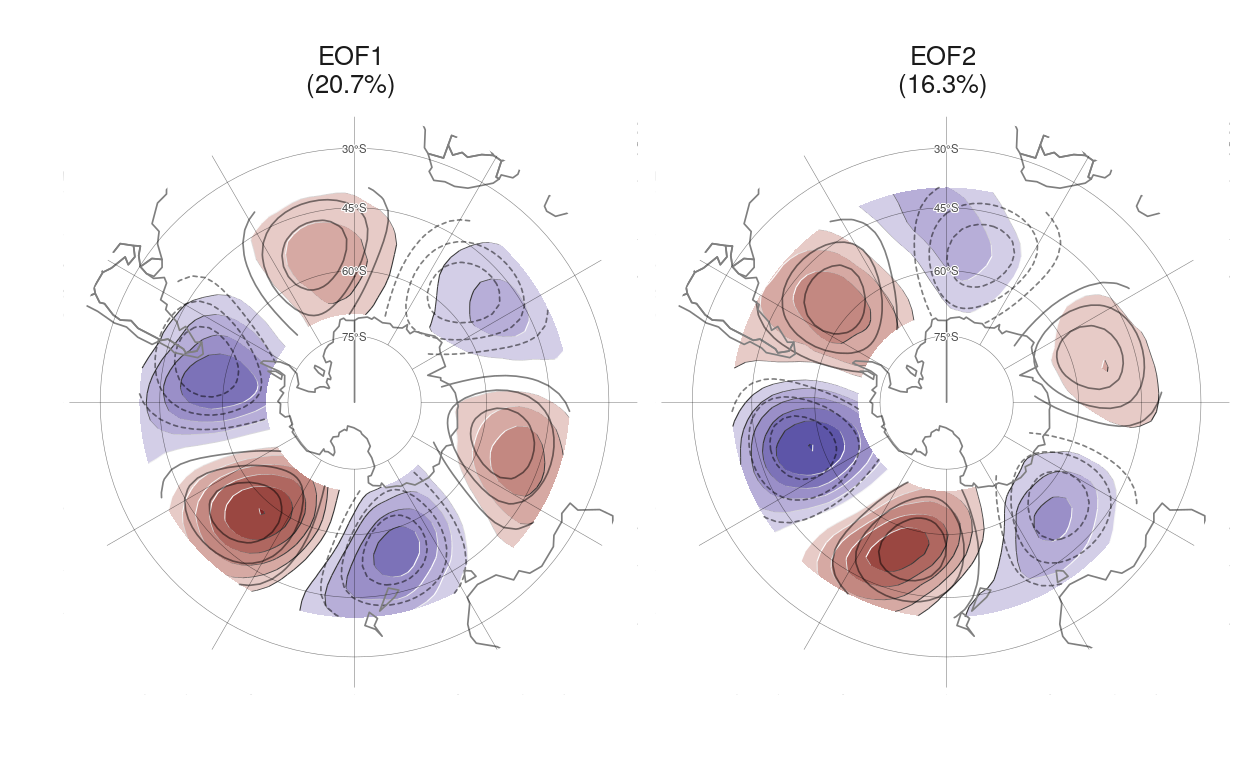# Comment on “A new zonal wave 3 index for the Southern Hemisphere”

How to measure a wave that is not just a wave.

Elio Campitelli https://eliocamp.github.io/ (Centro de Investigaciones del Mar y la Atmósfera)http://www.cima.fcen.uba.ar/
2022-06-2

My PhD protect is (ostensibly) about the zonal wave 3 (ZW3) in the Southern Hemisphere. A zonal wave 3 is a short way of saying that a wave with a wavelenth 1/3rd of the circumference of the Earth at that latitude; which in essense means that on each latitude circle there are 3 maximums and 3 minimums.

So how do you measure the activity of this kind of wave in the atmosphere? Traditionally, one would compute the Fourier spectrum of geopotential height (or meridional wind) at some latitude or latitude band and look at the amplitude and phase of the 3rd wavenumber. Alternatively, Raphael (2004) took the mean value of geopotential anomalies in the climatological location of the three maximums.

Both approaches have the problem that assume that the wave 3 is a perfectly homogeneous wave with constant amplitude and phase in all longitudes. Raphael (2004)’s index is even more restrictive. Because it’s based on fixed locations, it actually measures the amplitude of the wave 3 that is projected into the direction of the climatological wave (this might be an useful concept, though).

However, wave 3 activity is not such a clean wave at all. Many studies and simulations show that the atmospheric circulation that is wave 3-like is much more intense over the South Pacific than over the southern Indian Ocean and that the waves don’t stick to a constant latitude.

A recent paper by lead author Rishav Goyal proposes a different approach (which is very similar to my own, which will hopefully be available in a scientific journal near you soon). Instead of imposing the shape of the wave 3, Goyal et al. (2022) derive the suitable wave 3 pattern from the data using Empirical Orthogonal Functions.Figure 1: First two EOFs of meridional wind between 70°S and 40°S (shaded, positive values in red, negative in blue). The pure wave-3 field is shown in thin black contours. In parenthesis, the variance explained by each EOF. Compare with the top panel in Figure 2 of Goyal et al. (2022).

As you can see in Figure 1, the first two EOFs of meridional wind anomalies are basically a wave 3 pattern but with all the important deviations from a neat homogeneous wave that we want. The amplitude is greater in the Pacific Ocean than in the Indian Ocean, the phase changes with latitude and the centres are not exactly where they would be otherwise. This is essentially right, and something that is consistent with previous studies.

This is not the first time EOF was applied to the wave 3 pattern. Yuan and Li (2008) did the same, but importantly, they used only one EOF to characterise the zonal wave 3 when you need two. This kind of thing happens all the time in atmospheric sciences. Since EOFs can only capture standing oscillations, any pattern that moves needs two or more EOFs to be described.

Goyal et al. (2022) does use two of them and furthermore argues that since they are clearly the two orthogonal phases of a wave 3-like pattern, one can combine the time series of each EOF into a single wave-like index

$ZW3 = \sqrt{EOF1^2 + EOF2^2} \cos\left(\tan^{-1} \left ( \frac{EOF2}{EOF1} \right ) \right )$

So, basically, you get the magnitude of the overall activity of the wave 3 as $$\sqrt{EOF1^2 + EOF2^2}$$ and the phase –the location– with $$\tan^{-1} \left ( \frac{EOF2}{EOF1} \right )$$.

I think this very neat and the way to go. It combines the convenient mathematical formulation of a wave with the flexibility of describing something that is not a pure sine wave. But I think there’s a small detail that can be improved upon.

In theory, one can combine any two random time series that way, but the idea is that this works only if the two series actually represent the two orthogonal phases of a wave-like thing. So the validity of this new index relies heavily on the patterns seen in Figure 1 being orthogonal.

They certainly look to be, but in detail, I think that they are not exactly. If one plots the magnitude $$\sqrt{EOF1^1 + EOF^2}$$ (Figure 2 panel a) it looks like the amplitude changes spatially on a scale similar to the wavelength of the wave, particularly in the Indian sector. I think this is not ideal and shows that the patterns obtained with EOF are only approximately suitable for the task. The panel b in Figure 2 shows how I think it should look. The amplitude is modulated on a spatial scale larger than the wave itself, creating a smoother field.